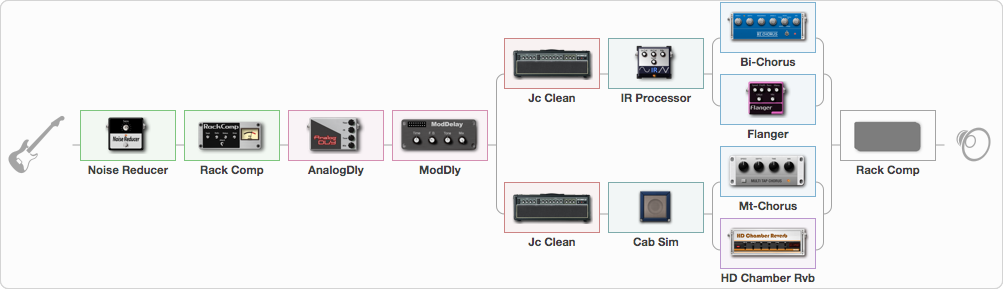# Aerial Diversion

Discussion in 'ToneLib-GFX presets' started by Trunk, Jun 13, 2020.

1. Aerial Diversion
Preset name: Aerial Diversion

Ambience... One for the Duritti Column fans... This preset is best employed with an open tuned acoustric guitar or an out of phase stratocaster, with an ostinato mid paced arpeggio or heavy consistant rhythm hand. Turning Off the Modulation splitter will give you a completely different wide open full field verb for subtle acoustic flare in a track. The Optional Rack Compressor at the end will dull the verb and effects and give the guitar more punch if needed. Turning off any post amp IR effect unit is designed deliberately to give a different soundscape... Hope it's inspiring!

Effects chain:Effect: "Noise Reducer" (Dynamics / Filter), active - "yes"
{

"Sens" = 50
"Mode" = Soft
}

Effect: "Rack Comp" (Dynamics / Filter), active - "yes"
{

"Threshold (dB)" = -23
"Ratio" = 5
"Attack" = Slow
"Release (ms)" = 250
"Knee" = 34
"Level (dB)" = 7
}

Effect: "AnalogDly" (Delay), active - "yes"
{

"Time" = Note sync, 1/8 Dotted
"Feedback" = 59
"Tone" = 50
"Mix" = 50
}

Effect: "ModDly" (Delay), active - "yes"
{

"Time" = Note sync, 1/4
"Feedback" = 39
"Tone" = 50
"Speed" = 1.3
"Mix" = 60
}

Effect: "Splitter" (Dynamics / Filter), active - "yes"
{

"A-Bypass" = Off
"A-Pan" = -30
"A-Level" = 60
"B-Bypass" = Off
"B-Pan" = 30
"B-Level" = 60
'A' branch:
{

Effect: "Jc Clean" (Amp simulators), active - "yes"
{

"Gain" = 30
"Bass" = 50
"Middle" = 60
"Treble" = 65
"Presence" = 65
"Master" = 81
"Level (dB)" = 4
}
Effect: "IR Processor" (Cabinets), active - "yes"
{

"IR" = Cab IR Sample4
"Low Cut (Hz)" = 0
"Hi Cut (kHz)" = 20.0
"Mix" = 100
"Level (dB)" = 4
}
Effect: "Splitter" (Dynamics / Filter), active - "yes"
{

"A-Bypass" = Off
"A-Pan" = -100
"A-Level" = 40
"B-Bypass" = Off
"B-Pan" = 0
"B-Level" = 79
'A' branch:
{

Effect: "Bi-Chorus" (Modulation / Sfx), active - "yes"
{

"Speed A" = 1.8
"Speed B" = 3.6
"Depth" = 71
"Reso" = 30
"Mode" = OppPhase
"Mix" = 50
}
}
'B' branch:
{

Effect: "Flanger" (Modulation / Sfx), active - "yes"
{

"Speed" = 0.4
"Depth" = 82
"Reso" = 52
"Center" = 6.4
"Offset" = 5.0
"Mix" = 65
"Reset" = Off
}
}
}
}
'B' branch:
{

Effect: "Jc Clean" (Amp simulators), active - "yes"
{

"Gain" = 30
"Bass" = 50
"Middle" = 60
"Treble" = 65
"Presence" = 65
"Master" = 81
"Level (dB)" = 4
}
Effect: "Cab Sim" (Cabinets), active - "yes"
{

"Model" = 2x12" Blackface
"Level (dB)" = 4
}
Effect: "Splitter" (Dynamics / Filter), active - "yes"
{

"A-Bypass" = Off
"A-Pan" = -80
"A-Level" = 55
"B-Bypass" = Off
"B-Pan" = 67
"B-Level" = 83
'A' branch:
{

Effect: "Mt-Chorus" (Modulation / Sfx), active - "yes"
{

"Speed" = 1.5
"Depth" = 92
"Time" = 7.7
"Mix" = 100
}
}
'B' branch:
{

Effect: "HD Chamber Rvb" (Reverb), active - "yes"
{

"Time" = 9.0
"PreLPF" = 79
"PreDelay" = 60
"HiDamp" = 21
"LoGain" = -2.1
"Mix" = 73
}
}
}
}
}

Effect: "Rack Comp" (Dynamics / Filter), active - "no"
{

"Threshold (dB)" = -9
"Ratio" = 5
"Attack" = Slow
"Release (ms)" = 90
"Knee" = 15
"Level (dB)" = 1
}

Note: You will need to download and install the ToneLib-GFX software to use the preset.

#### Attached Files:

• ###### Aerial_Diversion.tlgfx
File size:
5.1 KB
Views:
4,027
Last edited: Jun 14, 2020